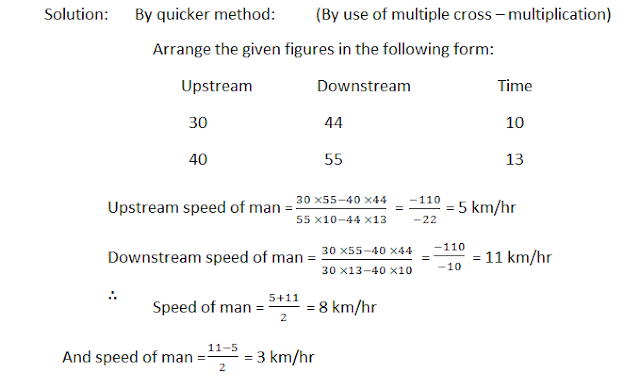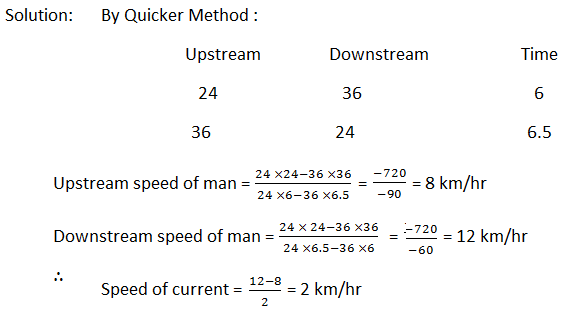# Boats and Streams Problems with Video Lecture

Normally by speed of the boat or swimmer we mean the speed of the boat (or swimmer) in still water. If the boat (or the swimmer) moves against the stream then it is called upstream and if it moves with the stream, it is called downstream.### Must watch this video lecture

==>> Get full video lecture DVDs

If the speed of the Boat (or swimmer) is x and if the speed of the stream is y then,
upstream the effective speed of the boat = x – y
and downstream the effective speed of the boat = x + y.
Theorem:If x km per hour be the man’s rate in still water, and y km per hour the rate of the current. Then
x+ y = man’s rate with current
x– y = man’s rate against current.
Adding and subtracting and then dividing by 2.
x = 1/2 (man’s rate with current + his rate against current)
y = 1/2 (man’s rate with current - his rate against current)

### Hence, we have the following two facts:

(i) A man’s rate in still water is half the sum of his rates with and against the current.
(ii) The rate of the current is half the difference between the rates of the man with and against the current.

#### Ques 1.

A man can row upstream at 10 km/hr and downstream at 16 km/hr. Find the man’s rate in still water and the rate of the current.
Solution: Rate in still water = 1/2 (10 + 16) = 13 km/hr
Rate of current = 1/2 (16 – 10) = 3km/hr.

#### Ques 2.

A man swims downstream 30 km and upstream 18 km, taking 3 hrs each time. What is the velocity of current?
Solution: Man’s rate downstream = 30/3 km/hr = 10 km/hr
Man’s rate upstream = 18/3 km/hr = 6 km/hr
∴ Velocity of stream = (10-6 )/2 = 2 km/hr

#### Ques 3.

A man can row 6 km/hr in still water. It takes him twice as long to row up as to row down the river. Find the rate of the stream.Method: II
We have, up rate + down rate = 2 × rate in still water
= 2 × 6 = 12 km/hr
Also, up rate : down rate = 1 : 2
So, dividing 12 in the ratio of 1 : 2, we get
Up rate = 4 km/hr
Down rate = 8 km/hr
∴ Rate of stream = (8-4 )/2 = 2 km/hr

Method III (shortest method)
Let rate of stream = x km/hr
Then, 6 + x = 2 (6 – x)
∴ x = 6/3 = 2 km/hr

#### Ques 4.

A man row 6 km/hr in still water. When the river is running at 1.2 km/hr, it takes him 1 hr to row to place and back. How far is the place?#### Ques 5.

A man can row 7 km/hr in still water. In a stream which is flowing at 3 km/hr, it takes him 7 hrs to row to a place and back. Hoe far is the place?#### Ques 6.

In a stream running at 2 km/hr, a motorboat goes 10 km upstream and back again to the starting point in 55 minutes. Find the speed of the motorboat in still water.#### Ques 7.

A man can row 30 km upstream and 44 km downstream in 10 hrs. Also, he can row 44 km upstream and 55 km downstream in 13 hrs. Find the rate of the current and the speed of the man in still water.#### Ques 8.

A boat covers 24 km upstream and 36 km downstream in 6 hrs, while it covers 36 km upstream and 24 km downstream in 6.5 hrs. find the velocity of the current.